# Mean State

Period Mean (original grids) [K]
Model Period Mean (intersection) [K]
Model Period Mean (complement) [K]
Benchmark Period Mean (intersection) [K]
Benchmark Period Mean (complement) [K]
Bias [K]
RMSE [K]
Phase Shift [months]
Bias Score 
RMSE Score 
Seasonal Cycle Score 
Spatial Distribution Score 
Interannual Variability Score 
Overall Score 
Benchmark [-] 299.
CLM4 [-] 298. 298. 300. 298. 300. -0.207 0.914 0.514 0.538 0.680 0.946 0.994 0.908 0.791
CLM4.5 [-] 298. 298. 300. 298. 300. -0.00920 0.830 0.455 0.575 0.710 0.958 0.999 0.930 0.814
CLM5 [-] 299. 299. 300. 298. 300. 0.285 0.690 0.366 0.667 0.779 0.967 1.00 0.955 0.858
Period Mean (original grids) [K]
Model Period Mean (intersection) [K]
Model Period Mean (complement) [K]
Benchmark Period Mean (intersection) [K]
Benchmark Period Mean (complement) [K]
Bias [K]
RMSE [K]
Phase Shift [months]
Bias Score 
RMSE Score 
Seasonal Cycle Score 
Spatial Distribution Score 
Interannual Variability Score 
Overall Score 
Benchmark [-] 300.
CLM4 [-] 300. 300. 300. 300. 300. 0.0637 0.861 0.221 0.788 0.783 0.983 0.991 0.936 0.877
CLM4.5 [-] 300. 300. 300. 300. 300. 0.276 0.853 0.188 0.758 0.825 0.986 0.986 0.955 0.889
CLM5 [-] 301. 301. 301. 300. 300. 0.473 0.802 0.168 0.746 0.862 0.987 0.990 0.964 0.902
Period Mean (original grids) [K]
Model Period Mean (intersection) [K]
Model Period Mean (complement) [K]
Benchmark Period Mean (intersection) [K]
Benchmark Period Mean (complement) [K]
Bias [K]
RMSE [K]
Phase Shift [months]
Bias Score 
RMSE Score 
Seasonal Cycle Score 
Spatial Distribution Score 
Interannual Variability Score 
Overall Score 
Benchmark [-] 295.
CLM4 [-] 295. 295. 296. 295. 297. 0.186 0.864 0.332 0.737 0.803 0.959 0.999 0.944 0.874
CLM4.5 [-] 295. 295. 296. 295. 297. 0.367 0.842 0.267 0.725 0.842 0.970 1.00 0.949 0.888
CLM5 [-] 296. 296. 296. 295. 297. 0.505 0.854 0.273 0.707 0.867 0.968 0.999 0.960 0.895
Period Mean (original grids) [K]
Model Period Mean (intersection) [K]
Model Period Mean (complement) [K]
Benchmark Period Mean (intersection) [K]
Benchmark Period Mean (complement) [K]
Bias [K]
RMSE [K]
Phase Shift [months]
Bias Score 
RMSE Score 
Seasonal Cycle Score 
Spatial Distribution Score 
Interannual Variability Score 
Overall Score 
Benchmark [-] 262.
CLM4 [-] 261. 261. 266. 262. 266. -0.321 1.44 0.0136 0.934 0.937 0.999 1.00 0.928 0.956
CLM4.5 [-] 261. 261. 266. 262. 266. -0.204 1.36 0.0125 0.938 0.940 0.999 1.00 0.930 0.958
CLM5 [-] 262. 261. 267. 262. 266. 0.258 1.13 0.0161 0.941 0.957 0.999 1.00 0.939 0.965
Period Mean (original grids) [K]
Model Period Mean (intersection) [K]
Model Period Mean (complement) [K]
Benchmark Period Mean (intersection) [K]
Benchmark Period Mean (complement) [K]
Bias [K]
RMSE [K]
Phase Shift [months]
Bias Score 
RMSE Score 
Seasonal Cycle Score 
Spatial Distribution Score 
Interannual Variability Score 
Overall Score 
Benchmark [-] 299.
CLM4 [-] 299. 299. 299. 299. 299. -0.368 0.940 0.699 0.312 0.586 0.895 0.938 0.906 0.704
CLM4.5 [-] 299. 299. 299. 299. 299. -0.197 0.851 0.662 0.357 0.619 0.900 0.932 0.907 0.722
CLM5 [-] 299. 299. 300. 299. 299. 0.226 0.750 0.486 0.425 0.725 0.930 0.963 0.913 0.780
Period Mean (original grids) [K]
Model Period Mean (intersection) [K]
Model Period Mean (complement) [K]
Benchmark Period Mean (intersection) [K]
Benchmark Period Mean (complement) [K]
Bias [K]
RMSE [K]
Phase Shift [months]
Bias Score 
RMSE Score 
Seasonal Cycle Score 
Spatial Distribution Score 
Interannual Variability Score 
Overall Score 
Benchmark [-] 271.
CLM4 [-] 270. 270. 274. 271. 274. -0.783 1.25 0.00895 0.936 0.943 0.999 1.00 0.973 0.966
CLM4.5 [-] 271. 270. 274. 271. 274. -0.735 1.22 0.00895 0.937 0.945 0.999 1.00 0.974 0.967
CLM5 [-] 271. 271. 275. 271. 274. -0.0249 0.647 0.00842 0.966 0.971 0.999 1.00 0.979 0.981
Period Mean (original grids) [K]
Model Period Mean (intersection) [K]
Model Period Mean (complement) [K]
Benchmark Period Mean (intersection) [K]
Benchmark Period Mean (complement) [K]
Bias [K]
RMSE [K]
Phase Shift [months]
Bias Score 
RMSE Score 
Seasonal Cycle Score 
Spatial Distribution Score 
Interannual Variability Score 
Overall Score 
Benchmark [-] 280.
CLM4 [-] 280. 280. 288. 280. 285. -0.256 1.33 0.0234 0.892 0.931 0.998 0.999 0.953 0.951
CLM4.5 [-] 280. 280. 288. 280. 285. -0.186 1.30 0.0230 0.894 0.934 0.998 0.999 0.954 0.952
CLM5 [-] 281. 280. 288. 280. 285. 0.249 1.09 0.0222 0.901 0.956 0.998 1.00 0.965 0.963
Period Mean (original grids) [K]
Model Period Mean (intersection) [K]
Model Period Mean (complement) [K]
Benchmark Period Mean (intersection) [K]
Benchmark Period Mean (complement) [K]
Bias [K]
RMSE [K]
Phase Shift [months]
Bias Score 
RMSE Score 
Seasonal Cycle Score 
Spatial Distribution Score 
Interannual Variability Score 
Overall Score 
Benchmark [-] 297.
CLM4 [-] 296. 296. 300. 297. 300. -0.473 1.33 0.200 0.747 0.817 0.980 0.998 0.937 0.883
CLM4.5 [-] 297. 297. 300. 297. 300. -0.254 1.23 0.191 0.756 0.837 0.981 0.999 0.940 0.892
CLM5 [-] 297. 297. 300. 297. 300. 0.110 1.08 0.181 0.766 0.877 0.983 1.00 0.946 0.908
Period Mean (original grids) [K]
Model Period Mean (intersection) [K]
Model Period Mean (complement) [K]
Benchmark Period Mean (intersection) [K]
Benchmark Period Mean (complement) [K]
Bias [K]
RMSE [K]
Phase Shift [months]
Bias Score 
RMSE Score 
Seasonal Cycle Score 
Spatial Distribution Score 
Interannual Variability Score 
Overall Score 
Benchmark [-] 264.
CLM4 [-] 263. 263. 266. 264. 264. -0.568 1.27 0.0103 0.946 0.940 0.999 1.00 0.953 0.963
CLM4.5 [-] 263. 263. 266. 264. 264. -0.485 1.14 0.0109 0.951 0.947 0.999 1.00 0.952 0.966
CLM5 [-] 264. 264. 267. 264. 264. 0.0517 0.717 0.0135 0.967 0.966 0.999 1.00 0.963 0.977
Period Mean (original grids) [K]
Model Period Mean (intersection) [K]
Model Period Mean (complement) [K]
Benchmark Period Mean (intersection) [K]
Benchmark Period Mean (complement) [K]
Bias [K]
RMSE [K]
Phase Shift [months]
Bias Score 
RMSE Score 
Seasonal Cycle Score 
Spatial Distribution Score 
Interannual Variability Score 
Overall Score 
Benchmark [-] 295.
CLM4 [-] 295. 295. 293. 295. 294. 0.233 0.671 0.0903 0.897 0.910 0.994 1.00 0.966 0.946
CLM4.5 [-] 295. 295. 293. 295. 294. 0.234 0.671 0.0898 0.896 0.911 0.994 1.00 0.967 0.946
CLM5 [-] 295. 295. 294. 295. 294. 0.479 0.699 0.0807 0.876 0.929 0.994 1.00 0.969 0.950
Period Mean (original grids) [K]
Model Period Mean (intersection) [K]
Model Period Mean (complement) [K]
Benchmark Period Mean (intersection) [K]
Benchmark Period Mean (complement) [K]
Bias [K]
RMSE [K]
Phase Shift [months]
Bias Score 
RMSE Score 
Seasonal Cycle Score 
Spatial Distribution Score 
Interannual Variability Score 
Overall Score 
Benchmark [-] 282.
CLM4 [-] 281. 281. 284. 282. 286. -0.398 1.19 0.130 0.880 0.900 0.991 0.997 0.952 0.937
CLM4.5 [-] 281. 281. 284. 282. 286. -0.410 1.16 0.127 0.879 0.905 0.991 0.999 0.957 0.939
CLM5 [-] 282. 282. 284. 282. 286. 0.117 0.866 0.145 0.899 0.936 0.990 1.00 0.970 0.955
Period Mean (original grids) [K]
Model Period Mean (intersection) [K]
Model Period Mean (complement) [K]
Benchmark Period Mean (intersection) [K]
Benchmark Period Mean (complement) [K]
Bias [K]
RMSE [K]
Phase Shift [months]
Bias Score 
RMSE Score 
Seasonal Cycle Score 
Spatial Distribution Score 
Interannual Variability Score 
Overall Score 
Benchmark [-] 287.
CLM4 [-] 282. 286. 248. 287. 292. -0.253 1.12 0.171 0.814 0.862 0.982 1.00 0.947 0.911
CLM4.5 [-] 282. 286. 248. 287. 292. -0.166 1.08 0.158 0.819 0.873 0.984 0.999 0.950 0.916
CLM5 [-] 283. 287. 249. 287. 292. 0.227 0.898 0.137 0.829 0.907 0.986 1.00 0.959 0.931
Period Mean (original grids) [K]
Model Period Mean (intersection) [K]
Model Period Mean (complement) [K]
Benchmark Period Mean (intersection) [K]
Benchmark Period Mean (complement) [K]
Bias [K]
RMSE [K]
Phase Shift [months]
Bias Score 
RMSE Score 
Seasonal Cycle Score 
Spatial Distribution Score 
Interannual Variability Score 
Overall Score 
Benchmark [-] 296.
CLM4 [-] 296. 296. 296. 296. 297. -0.135 0.977 0.177 0.891 0.916 0.987 0.999 0.958 0.944
CLM4.5 [-] 296. 296. 296. 296. 297. -0.0491 0.943 0.169 0.893 0.919 0.988 1.00 0.959 0.946
CLM5 [-] 296. 296. 296. 296. 297. 0.191 0.824 0.134 0.898 0.939 0.990 0.999 0.963 0.955
Period Mean (original grids) [K]
Model Period Mean (intersection) [K]
Model Period Mean (complement) [K]
Benchmark Period Mean (intersection) [K]
Benchmark Period Mean (complement) [K]
Bias [K]
RMSE [K]
Phase Shift [months]
Bias Score 
RMSE Score 
Seasonal Cycle Score 
Spatial Distribution Score 
Interannual Variability Score 
Overall Score 
Benchmark [-] 265.
CLM4 [-] 264. 264. 265. 265. 266. -0.421 1.39 0.0266 0.930 0.926 0.998 1.00 0.951 0.955
CLM4.5 [-] 264. 264. 265. 265. 266. -0.355 1.33 0.0209 0.933 0.929 0.999 1.00 0.949 0.956
CLM5 [-] 265. 265. 266. 265. 266. 0.255 0.978 0.0188 0.944 0.951 0.999 1.00 0.959 0.967
Period Mean (original grids) [K]
Model Period Mean (intersection) [K]
Model Period Mean (complement) [K]
Benchmark Period Mean (intersection) [K]
Benchmark Period Mean (complement) [K]
Bias [K]
RMSE [K]
Phase Shift [months]
Bias Score 
RMSE Score 
Seasonal Cycle Score 
Spatial Distribution Score 
Interannual Variability Score 
Overall Score 
Benchmark [-] 296.
CLM4 [-] 295. 295. 298. 296. 298. 0.162 1.19 0.237 0.676 0.838 0.980 0.998 0.946 0.879
CLM4.5 [-] 295. 295. 298. 296. 298. 0.156 1.19 0.224 0.675 0.833 0.982 0.996 0.952 0.878
CLM5 [-] 296. 295. 298. 296. 298. 0.401 1.19 0.241 0.667 0.862 0.979 0.997 0.945 0.885
Period Mean (original grids) [K]
Model Period Mean (intersection) [K]
Model Period Mean (complement) [K]
Benchmark Period Mean (intersection) [K]
Benchmark Period Mean (complement) [K]
Bias [K]
RMSE [K]
Phase Shift [months]
Bias Score 
RMSE Score 
Seasonal Cycle Score 
Spatial Distribution Score 
Interannual Variability Score 
Overall Score 
Benchmark [-] 286.
CLM4 [-] 286. 286. 293. 286. 292. -0.224 0.682 0.0272 0.940 0.948 0.998 1.00 0.976 0.969
CLM4.5 [-] 286. 286. 293. 286. 292. -0.150 0.647 0.0336 0.945 0.949 0.998 1.00 0.975 0.969
CLM5 [-] 286. 286. 294. 286. 292. 0.281 0.608 0.0307 0.942 0.960 0.998 1.00 0.973 0.972
Period Mean (original grids) [K]
Model Period Mean (intersection) [K]
Model Period Mean (complement) [K]
Benchmark Period Mean (intersection) [K]
Benchmark Period Mean (complement) [K]
Bias [K]
RMSE [K]
Phase Shift [months]
Bias Score 
RMSE Score 
Seasonal Cycle Score 
Spatial Distribution Score 
Interannual Variability Score 
Overall Score 
Benchmark [-] 275.
CLM4 [-] 274. 274. 277. 274. 278. -0.541 1.03 0.0552 0.925 0.938 0.996 0.996 0.970 0.961
CLM4.5 [-] 274. 274. 277. 274. 278. -0.508 0.990 0.0546 0.927 0.940 0.996 0.996 0.966 0.961
CLM5 [-] 274. 274. 278. 274. 278. 0.109 0.688 0.0418 0.943 0.959 0.997 0.999 0.972 0.971
Period Mean (original grids) [K]
Model Period Mean (intersection) [K]
Model Period Mean (complement) [K]
Benchmark Period Mean (intersection) [K]
Benchmark Period Mean (complement) [K]
Bias [K]
RMSE [K]
Phase Shift [months]
Bias Score 
RMSE Score 
Seasonal Cycle Score 
Spatial Distribution Score 
Interannual Variability Score 
Overall Score 
Benchmark [-] 284.
CLM4 [-] 283. 283. 287. 284. 286. 0.0193 1.17 0.0312 0.881 0.922 0.998 1.00 0.958 0.947
CLM4.5 [-] 283. 284. 287. 284. 286. 0.0333 1.19 0.0337 0.883 0.918 0.998 1.00 0.962 0.946
CLM5 [-] 284. 284. 287. 284. 286. 0.371 1.11 0.0268 0.879 0.936 0.998 1.00 0.963 0.952
Period Mean (original grids) [K]
Model Period Mean (intersection) [K]
Model Period Mean (complement) [K]
Benchmark Period Mean (intersection) [K]
Benchmark Period Mean (complement) [K]
Bias [K]
RMSE [K]
Phase Shift [months]
Bias Score 
RMSE Score 
Seasonal Cycle Score 
Spatial Distribution Score 
Interannual Variability Score 
Overall Score 
Benchmark [-] 299.
CLM4 [-] 299. 299. 300. 299. 299. -0.363 0.911 0.552 0.553 0.658 0.925 0.999 0.912 0.784
CLM4.5 [-] 299. 299. 299. 299. 299. -0.191 0.824 0.511 0.610 0.685 0.934 0.999 0.909 0.803
CLM5 [-] 299. 299. 300. 299. 299. 0.168 0.688 0.391 0.654 0.785 0.948 0.999 0.925 0.849
Period Mean (original grids) [K]
Model Period Mean (intersection) [K]
Model Period Mean (complement) [K]
Benchmark Period Mean (intersection) [K]
Benchmark Period Mean (complement) [K]
Bias [K]
RMSE [K]
Phase Shift [months]
Bias Score 
RMSE Score 
Seasonal Cycle Score 
Spatial Distribution Score 
Interannual Variability Score 
Overall Score 
Benchmark [-] 296.
CLM4 [-] 296. 296. 296. 296. 293. -0.0617 0.769 0.227 0.776 0.814 0.973 0.998 0.949 0.887
CLM4.5 [-] 296. 296. 296. 296. 293. 0.0743 0.703 0.246 0.785 0.833 0.971 0.997 0.952 0.895
CLM5 [-] 296. 296. 296. 296. 293. 0.383 0.666 0.220 0.763 0.880 0.973 1.00 0.963 0.910
Period Mean (original grids) [K]
Model Period Mean (intersection) [K]
Model Period Mean (complement) [K]
Benchmark Period Mean (intersection) [K]
Benchmark Period Mean (complement) [K]
Bias [K]
RMSE [K]
Phase Shift [months]
Bias Score 
RMSE Score 
Seasonal Cycle Score 
Spatial Distribution Score 
Interannual Variability Score 
Overall Score 
Benchmark [-] 284.
CLM4 [-] 284. 284. 288. 284. 285. 0.129 1.61 0.193 0.666 0.819 0.977 0.999 0.945 0.871
CLM4.5 [-] 284. 284. 288. 284. 285. 0.247 1.60 0.186 0.665 0.834 0.977 1.00 0.950 0.876
CLM5 [-] 285. 284. 288. 284. 285. 0.483 1.54 0.183 0.661 0.862 0.977 1.00 0.952 0.886

# Temporally integrated period mean

BENCHMARK MEAN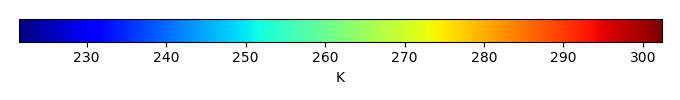MODEL MEANBIAS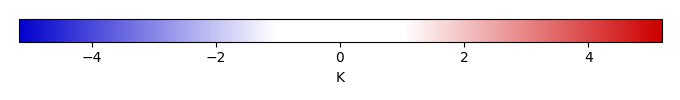BIAS SCORERMSE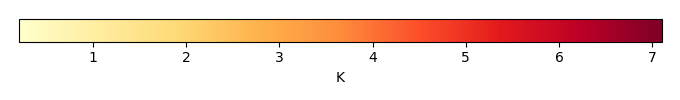RMSE SCOREBENCHMARK INTERANNUAL VARIABILITY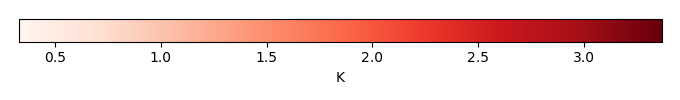MODEL INTERANNUAL VARIABILITYINTERANNUAL VARIABILITY SCOREBENCHMARK MAX MONTHMODEL MAX MONTHDIFFERENCE IN MAX MONTH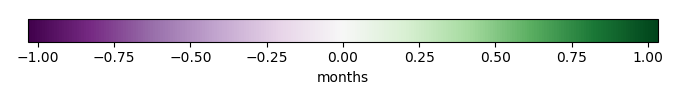SEASONAL CYCLE SCORESPATIAL TAYLOR DIAGRAMMODEL COLORS# Spatially integrated regional mean

MODEL COLORSREGIONAL MEANANNUAL CYCLEMONTHLY ANOMALYANNUAL CYCLE# All Models

BenchmarkCLM4CLM4.5CLM5# Data Information

creation_date: Fri Nov 1 11:52:30 PDT 2013

source_file: This product is generated from monthly CRU TS3.21 Mean Temperature observations

title: derived CRU high resolution (0.5x0.5) Mean Temperature

Approach: I simply read the monthly surface air temperature from original file, and separated the time series by a month and saved them by each month and each year.

Temporal resolution: monthly

General information: This product was derived from CRU v3.21 product.

Spatial resolution: 0.5x0.5 degree